# Speed and VelocityThe speed of a train is the distance it is traveling per unit of time. Its speed in a given direction is called its velocity. If the velocity of the train changes with time, the train is said to undergo acceleration or deceleration.

The speed of an object is the rate of motion of the object, or the rate at which the object changes its position. It is usually expressed as the distance d traveled by the object per unit of time t. Speed is a scalar quantity—it indicates how fast the object is traveling, but not the direction in which it is traveling.

The term velocity is used when one combines the speed of a moving object with the direction in which it is moving. Thus, velocity is a vector quantity, and speed may be regarded as the magnitude component of velocity. For example, the phrase "five meters per second" indicates the speed of the object, whereas the phrase "five meters per second east" indicates the velocity of the object.

## Contents

Vehicles often have a speedometer to measure their speed. When an object (such as an aircraft) is moving horizontally as well as vertically, a distinction is made between its "forward speed" and its "climbing speed."

## Measurement of speed and velocity

Both speed and velocity are measured in the same physical units, but speed does not contain the element of direction that velocity has.

The speed or velocity of an object is calculated by a simple mathematical formula:$v = \frac {d}{t}$

The average velocity (v) of an object moving a

where d is the distance moved by the object (or its "displacement") and t is the time it takes to move through that distance.

### Units

The dimensions for speed are distance/time.

In the SI (metric) system, it is measured in meters per second (m/s).

Units of speed include:

• meters per second, (symbol m/s), the SI derived unit
• kilometers per hour, (symbol kph or km/h)
• miles per hour, (symbol mph or mi/h)
• knots (nautical miles per hour, symbol kt)
• Mach, where Mach 1 is the speed of sound; Mach n is n times as fast.
Mach 1 ≈ 343 m/s ≈ 1235 km/h ≈ 768 mph (see the speed of sound for more detail)
• speed of light in vacuum (symbol c) is one of the natural units
c = 299,792,458 m/s
• the speed of sound in air is about 340 m/s, and 1500 m/s in water
• Other important conversions
1 m/s = 3.6 km/h
1 mph = 1.609 km/h
1 knot = 1.852 km/h = 0.514 m/s

## Average speed

Speed as a physical property represents primarily instantaneous speed. In real life we often use average speed (denoted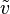$\tilde{v}$), which is rate of total distance (or length) and time interval. For example, if you go 60 miles in two hours, your average speed during that time is 60/2 = 30 miles per hour, but your instantaneous speed may have varied.

In mathematical notation: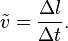$\tilde{v} = \frac{\Delta l}{\Delta t}.$

Instantaneous speed defined as a function of time on interval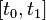$[t_0, t_1]$ gives average speed: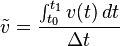$\tilde{v} = \frac{\int_{t_0}^{t_1} v(t) \, dt}{\Delta t}$

while instant speed defined as a function of distance (or length) on interval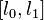$[l_0, l_1]$ gives average speed: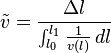$\tilde{v} = \frac{\Delta l}{\int_{l_0}^{l_1} \frac{1}{v(l)} \, dl}$

It is often intuitively expected, but incorrect, that going half a distance with speed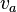$v_{a}$ and second half with speed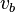$v_{b}$, produces total average speed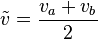$\tilde{v} = \frac{v_a + v_b}{2}$. The correct value is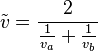$\tilde{v} = \frac{2}{\frac{1}{v_a} + \frac{1}{v_b}}$
(Note that the first is a proper arithmetic mean while the second is a proper harmonic mean).

Average speed can be derived also from speed distribution function (either in time or on distance):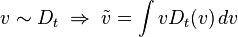$v \sim D_t\; \Rightarrow \; \tilde{v} = \int v D_t(v) \, dv$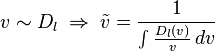$v \sim D_l\; \Rightarrow \; \tilde{v} = \frac{1}{\int \frac{D_l(v)}{v} \, dv}$

## Examples of different speeds

Below are some examples of different speeds (see also main article Orders of magnitude (speed)):

• Speed of a common snail = 0.001 m/s; 0.0036 km/h; 0.0023 mph.
• A brisk walk = 1.667 m/s; 6 km/h; 3.75 mph.
• Olympic sprinters (average speed over 100 meters) = 10 m/s; 36 km/h; 22.5 mph.
• Speed limit on a French autoroute = 36.111 m/s; 130 km/h; 80 mph.
• Top cruising speed of a Boeing 747-8 = 290.947 m/s; 1047.41 km/h; 650.83 mph.
• Official air speed record = 980.278 m/s; 3,529 km/h; 2,188 mph.
• Space shuttle on re-entry = 7,777.778 m/s; 28,000 km/h; 17,500 mph.

### Equations of motion

The instantaneous velocity vector (v) of an object that has position x(t), at time t, can be computed as the derivative:$v={\mathrm{d}x \over \mathrm{d}t} = \lim_{\Delta t \to 0}{\Delta x \over \Delta t}.$

The equation for an object's velocity can be obtained mathematically by taking the integral of the equation for its acceleration beginning from some initial period time$t_0$ to some point in time later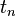$t_n$.

The final velocity v of an object which starts with velocity u and then accelerates at constant acceleration a for a period of time t is:$v = u + at.\;\!$

The average velocity of an object undergoing constant acceleration is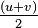$\begin{matrix} \frac {(u + v)}{2} \; \end{matrix}$, where u is the initial velocity and V is the final velocity. To find the displacement, s, of such an accelerating object during a time interval, t, then: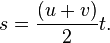$s = \frac {( u + v )}{2}t.$

When only the object's initial velocity is known, the expression,

also h=-16t2+vt+s

h is the height, v is the velocity, t is the time, and s is the starting height. Usually one will get two answers and must use logic to realize the real answer and what the other one pertains to.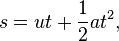$s = ut + \frac{1}{2}a t^2,$

can be used. These basic equations for final velocity and displacement can be combined to form an equation that is independent of time, also known as Torricelli's equation: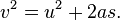$v^2 = u^2 + 2as.\,$

The above equations are valid for both classical mechanics and special relativity. Where classical mechanics and special relativity differ is in how different observers would describe the same situation. In particular, in classical mechanics, all observers agree on the value of t and the transformation rules for position create a situation in which all non-accelerating observers would describe the acceleration of an object with the same values. Neither is true for special relativity. In other words only relative velocity can be calculated.

The kinetic energy (energy of motion) of a moving object is linear with both its mass and the square of its velocity:$E_{K} = \begin{matrix} \frac{1}{2} \end{matrix} mv^2.$

The kinetic energy is a scalar quantity.

## V speeds

V speeds are speeds that define certain performance and limiting characteristics of an aircraft. (The "V" stands for velocity.) They are established by the manufacturer during design and testing and are specific to the aircraft model.

Usually, V speeds are relative to the air through which the aircraft is moving and are thus airspeeds. In many cases, they are defined by reference to the standard atmosphere or other specific conditions, taking into account the aircraft's maximum gross weight. The pilot is responsible for calculating the effective value based on the actual weight and air density. In other cases, the indicated airspeed, the value uncorrected for atmospheric pressure differences (height and temperature), is directly useful for the pilot.

In the U.S., V speeds are stated in knots or (for older aircraft models) miles per hour. For faster aircraft, some speeds are also defined by Mach number. Typically, V speeds are given for an aircraft at maximum gross weight, and are adjusted for lighter weights. The scheduled figures are given in the aircraft Flight Manual and Performance Manual.